• 粒子滤波算法原理及Matlab程序(专题).ppt》由会员分享，可在线阅读，更多相关《粒子滤波算法原理及Matlab程序(专题).ppt(18页珍藏版)》请在人人文库网上搜索。1、粒子滤波算法原理及Matlab程序,主讲： 方牛娃 QQ: ...

《粒子滤波算法原理及Matlab程序(专题).ppt》由会员分享，可在线阅读，更多相关《粒子滤波算法原理及Matlab程序(专题).ppt(18页珍藏版)》请在人人文库网上搜索。
1、粒子滤波算法原理及Matlab程序,主讲： 方牛娃 QQ: 345194112,1、粒子滤波的发展历史,90年代初，Gordon、Salmond、和Smith所提出的重采样(Resampling)技术 。 90年中期，计算机的计算能力的提高 近年来的新技术，EPF、UPF、RBPF等 新的应用领域：目标定位和跟踪、图像处理、语音处理、故障检测、经济数据处理,2、蒙特卡洛原理,粒子滤波技术是以蒙特卡洛为基础的 蒙特卡洛：用实验模拟的方法解决复杂的积分计算问题 硬币投掷实验(1) 掷一枚均匀硬币，正面朝上的次数X服从参数为，p的二项分布,XB(1,p) 在Matlab中编辑.m文件输入以下命令： 。
2、function cion_throw_test1 p=0.3; % 正面朝上的概率 m=1000; % 实验次数 fun(p,m); % 修改不同的p,m值 function fun(p,mm) pro=zeros(1,mm); randnum = binornd(1,p,1,mm); % 服从二项分布 a=0;,2、蒙特卡洛原理,蒙特卡洛的应用,应用说明：利用蒙特卡洛模拟计算圆周率 方法解决：,假设平面上有无数条距离为1的等距平行线，现向该平面随机投掷一根长度为l的针(l1)，则我们可计算该针与任一平行线相交的概率。这里，随机投针指的是：针的中心点与最近的平行线间的距离X均匀地分布在区间0。
3、,1/2上，针与平行线的夹角(不管相交与否)均匀的分布在区间0,上。此时，针与线相交的充要条件是,从而针线相交的概率为：,2、蒙特卡洛,% % 说明：利用蒙特卡洛模拟计算圆周率 % function buffon_test l=0.6; m=10000; % 实验次数 buffon(l,m); % function piguji=buffon(llength,mm) %llength 是针的长度 %mm 是随机实验次数 frq=0; xrandnum = unifrnd(0,0.5,1,mm); phi= unifrnd(0,pi,1,mm); for ii=1:mm if (xrandnum。
4、(1,ii)=(llength*sin(phi(1,ii)/2) frq=frq+1; end end piguji=2*llength/(frq/mm) 实验结果如下：,3、粒子滤波原理,粒子滤波目前有四大基本的重采样方法，分别是残差重采样(Residual resampling)，多项式重采样(Multinomial resampling)，系统重采样(Systematic resampling)，随机重采样(random resampling)，关于他们的原理，读者可以到网上检索相关的论文。,3、粒子滤波原理,随机重采样,执行仿真程序，得到以下仿真结果，图中上部就是程序中给定的W随机样本。
5、，而下部分是由随机采样得到的V样本集合。从连线关系可以看出，W样本集中的第2个样本被复制一次，第3个样本被复制2次，第7个样本被复制2次，第8个样本被复制1次，第9个样本被复制2次，W中除了被复制的样本外，其他样本被舍弃，这就是“优胜劣汰”的思想。,4、粒子滤波在单目标跟踪中的应用,状态方程： 观测方程： 噪声模型：Q、R,4、纯方位角单目标跟踪,纯方位跟踪系统仿真程序 % % 程序说明： 单站单目标基于角度的跟踪系统，采用粒子滤波算法 % 状态方程 X(k+1)=F*X(k)+Lw(k) % 观测方程 Z(k)=h(X)+v(k) function main % % 初始化参数 clear;。
6、 T=1; % 采样周期 M=30; % 采样点数 delta_w=1e-4; % 过程噪声调整参数，设得越大，目标运行的机动性越大，轨迹越随机(乱) Q=delta_w*diag(0.5,1,0.5,1) ; % 过程噪声均方差 R=pi/180*0.1; % 观测角度均方差，可将0.1设置的更小 F=1,T,0,0;0,1,0,0;0,0,1,T;0,0,0,1; % 系统初始化 % Length=100; % 目标运动的场地空间 Width=100; % 观测站的位置随即部署 Node.x=Width*rand; Node.y=Length*rand;,5、粒子滤波在多目标跟踪中的应用,。
7、多目标跟踪系统 状态方程 观测方程,5、粒子滤波在多目标跟踪中的应用,近邻法分类,5、粒子滤波在多目标跟踪中的应用,近邻法分类程序 % % 函数功能：近邻法分类程序 % function Neighbour_Classify_exam2 % 初始化数据 Type=3; % 假设已知三类目标 % 随机产生M个样本点 M=30; % 场地空间的长和宽 Width=4; Length=4; % % 样本初始化、并模拟一组样本空间 for i=1:Type x0=10*cos(pi*2*i/3); y0=10*sin(pi*2*i/3); XXi=x0,y0; % 已知类别的样本集合 for j=1:。
8、M % 模拟真实样本的空间，在类空间的位置 Xi(:,j)=x0+Width*randn;y0+Length*randn; end End ,5、粒子滤波在多目标跟踪的应用,基于近邻法的多目标跟踪粒子滤波程序 % % 单站多目标跟踪的建模程序，并用近邻法分类 % 主要模拟多目标的运动和观测过程，涉及融合算法-近邻法 function MTT_Model_With_NNClass_PF_SingleStation % % 初始化参数 % 观测站位置，随机的 T=10; % 仿真时间长度 TargetNum=3; % 目标个数 dt=1; % 采样时间间隔 S.x=100*rand; % 观测站水。
9、平位置 S.y=100*rand; % 观测站纵向位置 F=1,dt,0,0;0,1,0,0;0,0,1,dt;0,0,0,1; % 采用CV模型的状态转移矩阵 G=0.5*dt2,0;dt,0;0,0.5*dt2;0,dt; % 过程噪声驱动矩阵 H=1,0,0,0;0,0,1,0; % 观测矩阵,跟踪误差,跟踪轨迹,5、粒子滤波在电池寿命预测中的应用,状态方程 观测方程 原始实验数据(右图),5、粒子滤波在电池寿命预测中的应用,程序清单 % % 函数功能：粒子滤波用于电源寿命预测 function main % % 初始化 load Battery_Capacity % 运行程序时需要将B。
10、attery_Capacity.mat文件拷贝到程序所在文件夹 N=length(A12Cycle); % cycle的总数 M=200; % 粒子总数目 Future_Cycle=100; % 未来趋势 if N260 N=260; % 滤除大于260以后的数字 end,参考资料,目 录 第一部分 原理篇 1 第一章 概述 1 1.1 粒子滤波的发展历史 1 1.2 粒子滤波的优缺点 2 1.3 粒子滤波的应用领域 3 第二章 蒙特卡洛方法 4 2.1 概念和定义 4 2.2 蒙特卡洛模拟仿真程序 5 2.2.1硬币投掷实验(1) 5 2.2.2硬币投掷实验(2) 5 2.2.3古典概率实验。
11、 6 4.2.4几何概率模拟实验 7 2.2.5复杂概率模拟实验 7 2.3 蒙特卡洛理论基础 10 2.3.1大数定律 10 2.3.2中心极限定律 10 2.3.3蒙特卡洛的要点 11 2.4 蒙特卡洛方法的应用 13 2.4.1 Buffon实验及仿真程序 13 2.4.2 蒙特卡洛方法计算定积分的仿真程序 14 第三章 粒子滤波 19 3.1 粒子滤波概述 19 3.1.1 蒙特卡洛采样原理 19 3.1.2 贝叶斯重要性采样 20 3.1.3 序列重要性抽样(SIS)滤波器 20 3.1.4 Bootstrap/SIR滤波器 22 3.2 粒子滤波重采样方法实现程序 23 3.2.1。
12、 随机重采样程序 24 3.2.2 多项式重采样程序 25 3.2.3 系统重采样程序 26 3.2.4 残差重采样程序 27 3.3 粒子滤波原理 28 3.3.1 高斯模型下粒子滤波的实例程序 28,参考资料,第二部分 应用篇 33 第四章 粒子滤波在单目标跟踪中的应用 33 4.1 目标跟踪过程描述 33 4.2 单站单目标跟踪系统建模 34 4.3 单站单目标观测距离的系统及仿真程序 37 4.3.1 基于距离的系统模型 37 4.3.2 基于距离的跟踪系统仿真程序 38 4.4 单站单目标纯方位角度观测系统及仿真程序 43 4.4.1 纯方位目标跟踪系统模型 43 4.4.2 纯方位。
13、跟踪系统仿真程序 44 4.5 多站单目标纯方位角度观测系统及仿真程序 47 4.5.1 多站纯方位目标跟踪系统模型 47 4.5.2 多站纯方位跟踪系统仿真程序 48 第五章 粒子滤波在多目标跟踪中的应用 54 5.1 多目标跟踪系统建模 54 5.1.1 单站多目标跟踪系统建模 54 5.1.2 多站多目标跟踪系统建模 55 5.1.3 单站多目标线性跟踪系统的建模仿真程序 55 5.1.4 多站多目标非线性跟踪系统的建模仿真程序 57 5.2 多目标跟踪分类算法 61 5.2.1 多目标数据融合概述 61 5.2.2 近邻法分类算法及程序 62 5.2.3 近邻法用于目标跟踪中的航迹关联及算法程序 66 5.2.4 K-近邻法分类算法 69 5.3 粒子滤波用于多目标跟算法中的状态估计 70 5.3.1 原理介绍 70 5.3.2 基于近邻法的多目标跟踪粒子滤波程序 71 第六章 粒子滤波在电池寿命预测中的应用 76 6.1 概述 76 6.2 电池寿命预测的模型 78 6.3 基于粒子滤波的电池寿命预测仿真程序 81,谢谢大家。

展开全文• 粒子滤波算法原理及Matlab程序 主讲 方牛娃 QQ: 345194112 1粒子滤波的发展历史 90年代初GordonSalmond和Smith所提出的重采样Resampling技术 90年中期计算机的计算能力的提高 近年来的新技术EPFUPFRBPF等 新的应用...
• 粒子滤波算法原理及 Matlab 程序 主讲 方牛娃 QQ: 345194112 1 粒子滤波的发展历史 ? 90 年代初 Gordon Salmond 和 Smith 所提出的重采样 Resampling 技术 ? 90 年中期计算机的计算能力的提高 ? 近年来的新技术 EPF ...
• 粒子滤波在单目标跟踪多目标跟踪电池寿命预测中的应用-粒子滤波算法原理及在多目标跟踪中的应用（Matlab程序）.ppt 本帖最后由 huangxu_love 于 2013-7-26 12:50 编辑 推荐一本学习粒子滤波原理的好资料《粒子...matlab
• 90年代初，Gordon、Salmond、和Smith所提出的重采样（Resampling）技术 90年中期，计算机的计算能力的提高 近年来的新技术，EPF、UPF、RBPF等 新的应用领域：目标定位和跟踪、图像处理、语音处理、故障检测、经济...
• 一直都觉得粒子滤波是个挺牛的东西，每次试图看文献都被复杂的数学符号搞得看不下去。一个偶然的机会发现了Rob Hess(http://web.engr.oregonstate.edu/~hess/)实现的...从代码入手，一下子就明白了粒子滤波原理。根
转自：http://www.cnblogs.com/yangyangcv/archive/2010/05/23/1742263.html
一直都觉得粒子滤波是个挺牛的东西，每次试图看文献都被复杂的数学符号搞得看不下去。一个偶然的机会发现了Rob Hess(http://web.engr.oregonstate.edu/~hess/)实现的这个粒子滤波。从代码入手，一下子就明白了粒子滤波的原理。根据维基百科上对粒子滤波的介绍(http://en.wikipedia.org/wiki/Particle_filter)，粒子滤波其实有很多变种，Rob Hess实现的这种应该是最基本的一种，Sampling Importance Resampling (SIR)，根据重要性重采样。下面是我对粒子滤波实现物体跟踪的算法原理的粗浅理解：  1）初始化阶段-提取跟踪目标特征  该阶段要人工指定跟踪目标，程序计算跟踪目标的特征，比如可以采用目标的颜色特征。具体到Rob Hess的代码，开始时需要人工用鼠标拖动出一个跟踪区域，然后程序自动计算该区域色调(Hue)空间的直方图，即为目标的特征。直方图可以用一个向量来表示，所以目标特征就是一个N*1的向量V。  2）搜索阶段-放狗  好，我们已经掌握了目标的特征，下面放出很多条狗，去搜索目标对象，这里的狗就是粒子particle。狗有很多种放法。比如，a)均匀的放：即在整个图像平面均匀的撒粒子(uniform distribution)；b)在上一帧得到的目标附近按照高斯分布来放，可以理解成，靠近目标的地方多放，远离目标的地方少放。Rob Hess的代码用的是后一种方法。狗放出去后，每条狗怎么搜索目标呢？就是按照初始化阶段得到的目标特征(色调直方图，向量V)。每条狗计算它所处的位置处图像的颜色特征，得到一个色调直方图，向量Vi，计算该直方图与目标直方图的相似性。相似性有多种度量，最简单的一种是计算sum(abs(Vi-V)).每条狗算出相似度后再做一次归一化，使得所有的狗得到的相似度加起来等于1.  3）决策阶段  我们放出去的一条条聪明的狗向我们发回报告，“一号狗处图像与目标的相似度是0.3”,“二号狗处图像与目标的相似度是0.02”,“三号狗处图像与目标的相似度是0.0003”,“N号狗处图像与目标的相似度是0.013”...那么目标究竟最可能在哪里呢？我们做次加权平均吧。设N号狗的图像像素坐标是(Xn,Yn),它报告的相似度是Wn,于是目标最可能的像素坐标X = sum(Xn*Wn),Y = sum(Yn*Wn).  4）重采样阶段Resampling  既然我们是在做目标跟踪，一般说来，目标是跑来跑去乱动的。在新的一帧图像里，目标可能在哪里呢？还是让我们放狗搜索吧。但现在应该怎样放狗呢？让我们重温下狗狗们的报告吧。“一号狗处图像与目标的相似度是0.3”,“二号狗处图像与目标的相似度是0.02”,“三号狗处图像与目标的相似度是0.0003”,“N号狗处图像与目标的相似度是0.013”...综合所有狗的报告，一号狗处的相似度最高，三号狗处的相似度最低，于是我们要重新分布警力，正所谓好钢用在刀刃上，我们在相似度最高的狗那里放更多条狗，在相似度最低的狗那里少放狗，甚至把原来那条狗也撤回来。这就是Sampling Importance Resampling，根据重要性重采样(更具重要性重新放狗)。  (2)->(3)->(4)->(2)如是反复循环，即完成了目标的动态跟踪。  根据我的粗浅理解，粒子滤波的核心思想是随机采样+重要性重采样。既然我不知道目标在哪里，那我就随机的撒粒子吧。撒完粒子后，根据特征相似度计算每个粒子的重要性，然后在重要的地方多撒粒子，不重要的地方少撒粒子。所以说粒子滤波较之蒙特卡洛滤波，计算量较小。这个思想和RANSAC算法真是不谋而合。RANSAC的思想也是(比如用在最简单的直线拟合上)，既然我不知道直线方程是什么，那我就随机的取两个点先算个直线出来，然后再看有多少点符合我的这条直线。哪条直线能获得最多的点的支持，哪条直线就是目标直线。想法非常简单，但效果很好。 
展开全文• 粒子滤波算法原理和应用进行综述. 首先针对非线性非高斯系统的状态滤波问题, 阐述粒子滤波的原 理; 然后在分析采样- 重要性-重采样算法基础上, 讨论粒子滤波算法存在的主要问题和改进手段; 最后从概率密度...
• 本书系统介绍粒子滤波算法的基本原理和关键技术，针对标准粒子滤波算法存在的粒子退化、计算量大的缺点介绍了多种改进的粒子滤波算法，包括基于重要性密度函数选择的粒子滤波算法、基于重采样技术的粒子滤波算法、...
• 粒子滤波算法原理和应用进行综述.首先针对非线性非高斯系统的状态滤波问题,阐述粒子滤波的原理;然后在分析采样-重要性-重采样算法基础上,讨论粒子滤波算法存在的主要问题和改进手段;最后从概率密度函数的角度...
• 粒子滤波算法原理和应用进行综述’首先针对非线性非高斯系统的状态滤波问题C阐述粒子滤波的原理D，很适合初学者使用。
• 今天我们来讲一下粒子滤波算法，这也是我在学习过程中的一个笔记，由于有很多的个人理解，有不对的地方欢迎大家批评指正。 另：个人博客 Glooow 开业啦！欢迎各位大驾光临 文章目录贝叶斯滤波1. 预测2.更新...
今天我们来讲一下粒子滤波算法，这也是我在学习过程中的一个笔记，由于有很多的个人理解，有不对的地方欢迎大家批评指正。

另：个人博客 Glooow 开业啦！欢迎各位大驾光临

文章目录
贝叶斯滤波1. 预测2.更新总结
卡尔曼滤波大数定律粒子滤波简单实例1. 预测2. 更新3. 递推重采样
粒子滤波原理推导总结Reference

贝叶斯滤波
贝叶斯滤波是我们理解粒子滤波的基础。假设我们有如下图的隐马尔科夫模型(Hidden Markov Model)，并且有如下的系统方程与观测方程，我们只有观测值

y

1

:

T

\boldsymbol{y}_{1:T}

，但是现在想要根据观测值估计隐变量

x

1

:

T

\boldsymbol{x}_{1:T}

，也就是滤波所要做的事情。

System Model

:

x

k

=

f

k

(

x

k

−

1

,

v

k

−

1

)

Observation Model

:

y

k

=

h

k

(

x

k

,

n

k

)

\begin{aligned} \text{System Model}&: x_{k}=f_{k}\left(x_{k-1}, v_{k-1}\right) \\ \text{Observation Model}&: \mathrm{y}_{k}=h_{k}\left(\mathrm{x}_{k}, \mathrm{n}_{k}\right) \end{aligned}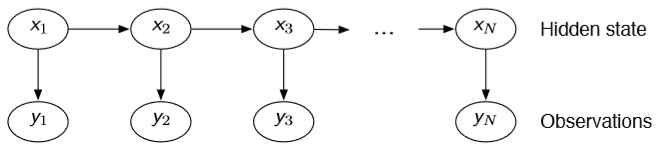假设我们现在已经处于

t

k

−

1

t_{k-1}

，且已经获得了

p

(

x

k

−

1

∣

y

1

:

k

−

1

)

p({x}_{k-1}|\boldsymbol{y}_{1:k-1})

，这个概率分布的含义是什么呢？我们现在已经有了前

k

−

1

k-1

个时刻的观测值

y

1

:

k

−

1

\boldsymbol{y}_{1:k-1}

，并且根据这些观测值估计了

k

−

1

k-1

时刻隐变量

x

k

−

1

x_{k-1}

的后验概率分布，也即

p

(

x

k

−

1

∣

y

1

:

k

−

1

)

p({x}_{k-1}|\boldsymbol{y}_{1:k-1})

。那么接下来到

k

k

时刻，我们又获得了一个观测

y

k

y_k

，要怎么估计新的后验概率分布

p

(

x

k

∣

y

1

:

k

)

p({x}_{k}|\boldsymbol{y}_{1:k})

呢？我们通过预测和更新两个阶段来估计，下面我就解释一下这两个阶段的含义。
1. 预测
前面说了我们有系统模型和观测模型，首先根据系统模型，我们有了

k

−

1

k-1

时刻的后验，就可以根据

x

k

−

1

x_{k-1}

到

x

k

x_k

的转移模型得到

x

k

x_k

对应的概率分布，这个过程就叫做预测(Update)。通过预测阶段，我们可以获得先验概率分布(注意这里我们将

p

(

x

k

∣

y

1

:

k

−

1

)

p({x}_k|\boldsymbol{y}_{1:k-1})

称为先验概率分布)

p

(

x

k

∣

y

1

:

k

−

1

)

=

∫

p

(

x

k

∣

x

k

−

1

)

p

(

x

k

−

1

∣

y

1

:

k

−

1

)

d

x

k

−

1

\begin{aligned}p({x}_k|\boldsymbol{y}_{1:k-1})=\int p({x}_k|x_{k-1})p(x_{k-1}|\boldsymbol{y}_{1:k-1})dx_{k-1}\end{aligned}

也就是说，我们即使没有观测值，也能对

x

k

x_k

的分布进行估计，但是由于没有利用观测值

y

k

y_k

带来的信息，因此这个估计是不准确的，下面我们就需要用观测值对这个先验概率分布进行纠正，也就是 更新阶段。
2.更新
有了先验，又有了观测，根据贝叶斯公式，我们可以很容易得到后验概率分布

p

(

x

k

∣

y

1

:

k

)

p\left(x_{k} | \boldsymbol{y}_{1: k}\right)

。怎么理解下面一个式子呢？我们有似然函数

p

(

y

k

∣

x

k

)

p\left(y_{k} | x_{k}\right)

，现在有了观测

y

k

y_k

，那么似然值越大，表明对应的

x

k

x_k

的概率应该也越大， 就是用似然函数对先验概率进行加权就得到了后验概率。

p

(

x

k

∣

y

1

:

k

)

=

p

(

y

k

∣

x

k

,

y

1

:

k

−

1

)

p

(

x

k

∣

y

1

:

k

−

1

)

p

(

y

k

∣

y

1

:

k

−

1

)

∝

p

(

y

k

∣

x

k

,

y

1

:

k

−

1

)

p

(

x

k

∣

y

1

:

k

−

1

)

=

p

(

y

k

∣

x

k

)

p

(

x

k

∣

y

1

:

k

−

1

)

\begin{aligned} p\left(x_{k} | \boldsymbol{y}_{1: k}\right)&=\frac{p\left(y_{k} | x_{k},\boldsymbol{y}_{1: k-1}\right) p\left(x_{k} | \boldsymbol{y}_{1: k-1}\right)}{p\left(y_{k} | \boldsymbol{y}_{1: k-1}\right)} \\ &\propto p\left(y_{k} | x_{k},\boldsymbol{y}_{1: k-1}\right) p\left(x_{k} | \boldsymbol{y}_{1: k-1}\right) \\ &= p\left(y_{k} | x_{k}\right) p\left(x_{k} | \boldsymbol{y}_{1: k-1}\right) \end{aligned}

怎么理解这个加权呢？举个栗子
比如

总结
总结起来，我们滤波分为两个阶段

Prediction

:

p

(

x

k

∣

y

1

:

k

−

1

)

=

∫

p

(

x

k

∣

x

k

−

1

)

p

(

x

k

−

1

∣

y

1

:

k

−

1

)

d

x

k

−

1

Update

:

p

(

x

k

∣

y

1

:

k

)

∝

p

(

y

k

∣

x

k

)

p

(

x

k

∣

y

1

:

k

−

1

)

\begin{aligned} \text{Prediction}&: p({x}_k|\boldsymbol{y}_{1:k-1})=\int p({x}_k|x_{k-1})p(x_{k-1}|\boldsymbol{y}_{1:k-1})dx_{k-1} \\ \text{Update}&: p\left(x_{k} | \boldsymbol{y}_{1: k}\right)\propto p\left(y_{k} | x_{k}\right) p\left(x_{k} | \boldsymbol{y}_{1: k-1}\right) \end{aligned}

可以看到，上面的预测阶段含有积分，这在实际当中往往是很难计算的，而对于那些非常规的PDF，甚至不能给出解析解。解决这种问题一般有两种思路：
建立简单的模型，获得解析解，如卡尔曼滤波(Kalman Filter)及EKF、UKF等；建立复杂的模型，获得近似解，如粒子滤波(Particle Filter)等。
卡尔曼滤波
卡尔曼滤波的思路就是将系统建模线性模型，隐变量、控制变量、控制噪声与观测噪声均为高斯分布，那么观测变量也是随机变量，整个模型中所有的随机变量都是高斯的！高斯分布是我们最喜欢的分布，因为在前面贝叶斯滤波的预测阶段我们可以不用积分了，只需要计算均值和协方差就可以了！根据系统模型和观测模型，我们可以获得滤波的闭式解，这就是卡尔曼滤波的思想。

System Model

:

x

k

=

A

x

k

−

1

+

B

u

k

−

1

+

v

k

−

1

Observation Model

:

y

k

=

C

x

k

+

n

k

\begin{aligned} \text{System Model}&: \mathrm{x}_{k}=A\mathrm{x}_{k-1} + B\mathrm{u}_{k-1} + v_{k-1} \\ \text{Observation Model}&: \mathrm{y}_{k}=C\mathrm{x}_{k}+ \mathrm{n}_{k} \end{aligned}

但实际中要求线性系统模型往往是很困难的，对于非线性模型，如果我们还想应用卡尔曼滤波该怎么办呢？线性近似，也就是求一个雅可比矩阵(Jacobi)，这就是扩展卡尔曼滤波(EKF)。
大数定律
粒子滤波是什么是意思呢？我们可以用大量的采样值来描述一个概率分布，当我们按照一个概率分布进行采样的时候，某个点的概率密度越高，这个点被采到的概率越大，当采样数目足够大(趋于无穷)的时候，粒子出现的频率就可以用来表示对应的分布，实际中我们可以理解一个粒子就是一个采样。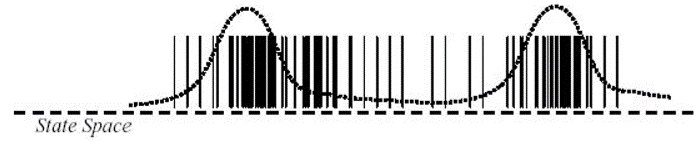但是对于离散型的随机变量还好，可以很容易的求出来状态空间中每个状态的频率。但如果对于连续分布，其实是很不方便的，所幸实际中我们也不需要获得概率分布的全部信息，一般只需要求出期望就可以了。
下面为了公式书写和推导方便，以离散型随机变量为例，假设状态空间为

Z

=

{

1

,

2

,

.

.

.

,

K

}

\mathcal{Z}=\{1,2,...,K\}

，有

N

N

个采样样本

z

i

∈

Z

z_i \in \mathcal{Z}

，服从概率分布

q

(

z

)

q(\mathbf{z})

。我们将根据

N

N

个采样样本

z

1

:

N

\boldsymbol{z}_{1:N}

得到的分布记为经验分布

p

^

(

b

∣

z

1

:

N

)

=

1

N

∑

i

I

(

b

−

z

i

)

\hat{p}(b|\boldsymbol{z}_{1:N}) = \frac{1}{N}\sum_i \mathbb{I}(b-z_i)

，其中

I

(

⋅

)

\mathbb{I}(\cdot)

为指示函数，那么当

N

N

足够大的时候，经验分布

p

^

(

b

∣

z

1

:

N

)

\hat{p}(b|\boldsymbol{z}_{1:N})

就足够接近真实分布

q

(

z

)

q(\mathbf{z})

。现在我们想估计随机变量

t

=

g

(

z

)

\mathsf{t}=g(\mathsf{z})

的期望，即

E

[

t

]

=

E

[

g

(

z

)

]

=

∑

b

∈

Z

g

(

b

)

q

(

b

)

≈

∑

b

g

(

b

)

p

^

(

b

∣

z

1

:

N

)

=

∑

b

g

(

b

)

1

N

∑

i

I

(

b

−

z

i

)

=

1

N

∑

i

g

(

z

i

)

\begin{aligned} \mathbb{E}[\mathsf{t}] &= \mathbb{E}[g(\mathsf{z})]=\sum_{b\in\mathcal{Z}}g(b)q(b) \\ &\approx \sum_b g(b)\hat{p}(b|\boldsymbol{z}_{1:N}) \\ &= \sum_b g(b)\frac{1}{N}\sum_i \mathbb{I}(b-z_i)\\ &= \frac{1}{N}\sum_i g(z_i) \end{aligned}

也就是说，我们只需要对样本进行简单求和就可以了！连续型随机变量也是类似的，所以在后面的粒子滤波中，我们利用粒子估计了概率密度分布，要想求期望就只需要进行求和平均就可以了。
粒子滤波简单实例
好了，有了前面的预备知识，我们可以先看一个粒子滤波的简单例子。
假设我们现在有采样好的

N

N

个粒子

{

x

k

−

1

i

}

i

=

1

N

\{x_{k-1}^i\}_{i=1}^N

，他们服从分布

p

(

x

k

−

1

∣

y

1

:

k

−

1

)

p({x}_{k-1}|\boldsymbol{y}_{1:k-1})

，那么我们现在如何进行贝叶斯滤波中的预测和更新阶段呢？
1. 预测
回顾一下预测的公式

Prediction

:

p

(

x

k

∣

y

1

:

k

−

1

)

=

∫

p

(

x

k

∣

x

k

−

1

)

p

(

x

k

−

1

∣

y

1

:

k

−

1

)

d

x

k

−

1

\text{Prediction}: p({x}_k|\boldsymbol{y}_{1:k-1})=\int p({x}_k|x_{k-1})p(x_{k-1}|\boldsymbol{y}_{1:k-1})dx_{k-1} \\

想一下：只要我们让每个粒子

x

k

−

1

i

x_{k-1}^i

“进化”一步，也就是说按照分布

p

(

x

k

∣

x

k

−

1

)

p(x_k|x_{k-1})

进行采样，使得

x

k

i

∼

p

(

x

k

∣

x

k

−

1

i

)

x_k^i \sim p(x_k|x_{k-1}^i)

，这样我们就获得了

N

N

个新的粒子

{

x

k

i

}

i

=

1

N

\{x_k^i\}_{i=1}^N

。很容易验证，如果

{

x

k

−

1

i

}

i

=

1

N

\{x_{k-1}^i\}_{i=1}^N

能够很好的表示

p

(

x

k

−

1

∣

y

1

:

k

−

1

)

p({x}_{k-1}|\boldsymbol{y}_{1:k-1})

的话，那么

{

x

k

i

}

i

=

1

N

\{x_k^i\}_{i=1}^N

也能很好的表示

p

(

x

k

∣

y

1

:

k

−

1

)

p({x}_k|\boldsymbol{y}_{1:k-1})

(这一部分可以凭直观感觉来理解，这是一个很自然的事情，也可以用前面的经验分布的思路进行公式推导)。
这样做的好处是什么呢？我们避免了积分！只需要对一个已知的分布

p

(

x

k

∣

x

k

−

1

i

)

p(x_k|x_{k-1}^i)

进行采样，而这个分布是我们假设的系统模型，可以是很简单的，因此采样也很方便。
2. 更新
通过预测我们获得了

x

k

i

∼

p

(

x

k

∣

x

k

−

1

i

)

x_k^i \sim p(x_k|x_{k-1}^i)

，这

N

N

个粒子就描述了先验概率分布。接下来就是更新，再回顾一下更新的公式

Update

:

p

(

x

k

∣

y

1

:

k

)

∝

p

(

y

k

∣

x

k

)

p

(

x

k

∣

y

1

:

k

−

1

)

\text{Update}: p\left(x_{k} | \boldsymbol{y}_{1: k}\right)\propto p\left(y_{k} | x_{k}\right) p\left(x_{k} | \boldsymbol{y}_{1: k-1}\right)

更新是什么呢？前面提到了：更新就是用似然函数对先验概率进行加权就得到了后验概率。如果简单的把

x

k

i

x_k^i

带入到上面的公式里，就可以得到下面的式子

p

(

x

k

i

∣

y

1

:

k

)

∝

p

(

y

k

∣

x

k

i

)

p

(

x

k

i

∣

y

1

:

k

−

1

)

p\left(x_{k}^i | \boldsymbol{y}_{1: k}\right)\propto p\left(y_{k} | x_{k}^i\right) p\left(x_{k}^i | \boldsymbol{y}_{1: k-1}\right)

实际上就表示每个粒子有不同的权重。

这里怎么理解呢？想一下前面提到的经验分布，我们只需要用粒子出现的频率来表示对应的概率，实际上就是在统计频率的时候每个粒子的权重都是相同的，出现一次计数就加一。
而这里的区别是什么呢？由于我们有一个观测值

y

k

y_k

，根据

y

k

y_k

来反推，某一个粒子

i

1

i_1

的导致

y

k

y_k

出现的概率更大，那么我们在统计频率的时候，就给这个粒子加一个更大的权重，以表示我们更相信/重视这个粒子。

那么问题来了，前面我们说了滤波过程中要想求期望，只需要简单求和就可以了

E

[

t

]

=

1

N

∑

i

g

(

z

i

)

\mathbb{E}[\mathsf{t}] = \frac{1}{N}\sum_i g(z_i)

现在粒子有了权重怎么办呢，同理，加个权就好了(推导也很简单，相信大家都会)，下面的式子里对权重进行了归一化

E

[

t

]

=

∑

i

w

i

∑

j

w

j

g

(

z

i

)

\mathbb{E}[\mathsf{t}] = \sum_i \frac{w_i}{\sum_j w_j}g(z_i)

3. 递推
前面只讲了一次预测和一次更新的过程，注意到我们前面只是从

k

−

1

k-1

到

k

k

时刻的滤波过程，但每一轮循环原理都是相同的。
不过细心的朋友们可能会觉得不太对劲，前面一个阶段的例子里，预测之前我们有采样

{

x

k

−

1

i

}

i

=

1

N

\{x_{k-1}^i\}_{i=1}^N

，推导过程中我们是默认这些粒子的权重都是相同的，然后我们进行了预测和更新，但是更新之后我们给每个粒子加权了呀，到下一个阶段怎么办？！！！也很简单，预测阶段不必要求每个粒子的权重都相同，也加上一个权重就好了。也就是说我们时时刻刻都保存有每个粒子的权重信息。
这样我们就可以得到一个完整的粒子滤波算法了！但是还有问题！
重采样
但是呢，有人发现了上面的算法不好啊！不是因为麻烦，而是滤波到最后会发现只有少数一部分粒子有很大的权重，而其他绝大部分粒子的权重几乎为 0，这就意味着对于那些“不重要”的粒子，他们在我们求期望的时候贡献并不大，但是我们却花费了大量的计算精力来维护这些粒子，这样不好不好！于是就有人提出了重采样。
重采样什么意思呢？你们粒子的权重不是不同吗，那我就采取手段给你们整相同了！简单理解，有两个粒子，粒子

a

a

的权重是 8，粒子

b

b

权重是 2，那我就把粒子

a

a

复制 8 份，粒子

b

b

复制 2 份，这样得到的 10 个粒子权重就都是 1 了。但是另一个问题是一开始我们只有 2 个粒子，这样弄完我们就有 10 个粒子了，如果一直这么做我们的粒子数会越来越多，算力跟不上。那就从这 10 个粒子里均匀分布地随机抽 2 个！那么粒子

a

a

的概率就是

0.8

0.8

，这就成了。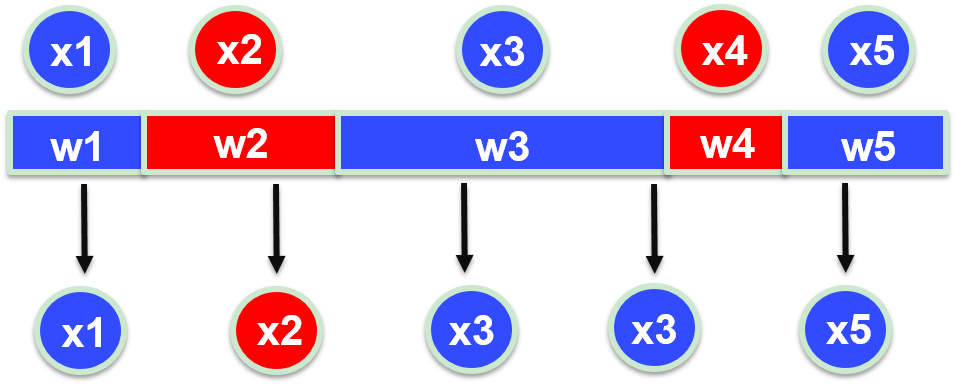至此，加上重采样我们就获得了一个完整的比较好用的粒子滤波算法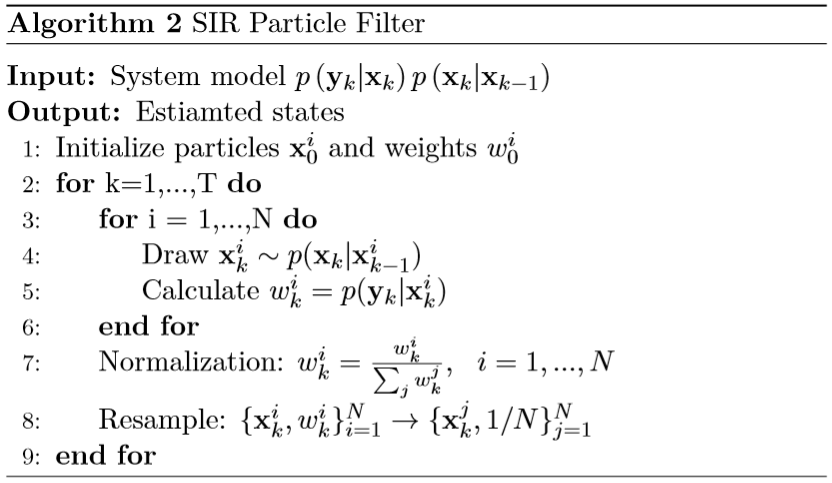粒子滤波原理推导
但是还有一个问题，就是前面我们直接说有

N

N

个粒子

x

k

−

1

i

∼

p

(

x

k

−

1

∣

y

1

:

k

−

1

)

x_{k-1}^i \sim p({x}_{k-1}|\boldsymbol{y}_{1:k-1})

，但是第 1 步(刚开始)的时候我们怎么获得这些粒子来描述

p

(

x

1

∣

y

1

)

p(x_1|y_1)

啊？如果是高斯分布还好，我们可以很方便的对高斯分布进行采样，但是如果是一个特别特别特别复杂的分布呢？我们甚至不能写出解析表达式，更没办法按照这个分布进行随机采样，那我们是不是就凉了？不！我们前面不是讲了粒子可以有权重嘛。
假如我们现在有另一个分布

q

(

x

1

:

k

∣

y

1

:

k

)

q(\mathbf{x}_{1:k}|\mathbf{y}_{1:k})

，这个分布是我们自己指定的，可以很简单，而且我们可以按照这个分布来进行采样，那么我们就有

x

k

−

1

i

∼

q

(

x

1

:

k

∣

y

1

:

k

)

x_{k-1}^i \sim q(\mathbf{x}_{1:k}|\mathbf{y}_{1:k})

，那么我们怎么用这些粒子来表示我们想要得到的真正的分布

p

(

x

0

:

k

∣

y

1

:

k

)

p(\mathbf{x}_{0:k}|\mathbf{y}_{1:k})

呢？再加个权就行了，就像前面用似然函数进行加权。

p

(

x

0

:

k

∣

y

1

:

k

)

≈

∑

i

=

1

N

w

k

i

δ

(

x

0

:

k

−

x

0

:

k

i

)

w

k

i

∝

p

(

x

0

:

k

i

∣

y

1

:

k

)

q

(

x

0

:

k

i

∣

y

1

:

k

)

\begin{aligned} p(\mathbf{x}_{0:k}|\mathbf{y}_{1:k})&\approx \sum_{i=1}^{N}w_k^i\delta(\mathbf{x}_{0:k}-\mathbf{x}_{0:k}^i) \\ w_{k}^{i} &\propto \frac{p\left(\mathbf{x}_{0: k}^{i} | \mathbf{y}_{1: k}\right)}{q\left(\mathbf{x}_{0: k}^{i} | \mathbf{y}_{1: k}\right)} \end{aligned}

再考虑前面提到的的预测和更新两个递推进行的阶段，就有

q

(

x

0

:

k

∣

y

1

:

k

)

=

q

(

x

k

∣

x

0

:

k

−

1

,

y

1

:

k

)

q

(

x

0

:

k

−

1

∣

y

1

:

k

−

1

)

=

q

(

x

k

∣

x

k

−

1

,

y

k

)

q

(

x

0

:

k

−

1

∣

y

1

:

k

−

1

)

w

k

i

∝

w

k

−

1

i

p

(

y

k

∣

x

k

i

)

p

(

x

k

i

∣

x

k

−

1

i

)

q

(

x

k

i

∣

x

k

−

1

i

,

y

k

)

\begin{aligned}q\left(\mathbf{x}_{0: k} | \mathbf{y}_{1: k}\right)&=q\left(\mathbf{x}_{k} | \mathbf{x}_{0: k-1}, \mathbf{y}_{1: k}\right) q\left(\mathbf{x}_{0: k-1} | \mathbf{y}_{1: k-1}\right) \\ &= q\left(\mathbf{x}_{k} | \mathbf{x}_{k-1}, \mathbf{y}_{ k}\right) q\left(\mathbf{x}_{0: k-1} | \mathbf{y}_{1: k-1}\right) \\ w_{k}^{i} &\propto w_{k-1}^{i} \frac{p\left(\mathbf{y}_{k} | \mathbf{x}_{k}^{i}\right) p\left(\mathbf{x}_{k}^{i} | \mathbf{x}_{k-1}^{i}\right)}{q\left(\mathbf{x}_{k}^{i} | \mathbf{x}_{k-1}^{i}, \mathbf{y}_{k}\right)} \end{aligned}

然后我们就得到了一个更加 universal/generic 的粒子滤波算法(下面图片所示算法中没加重采样步骤)。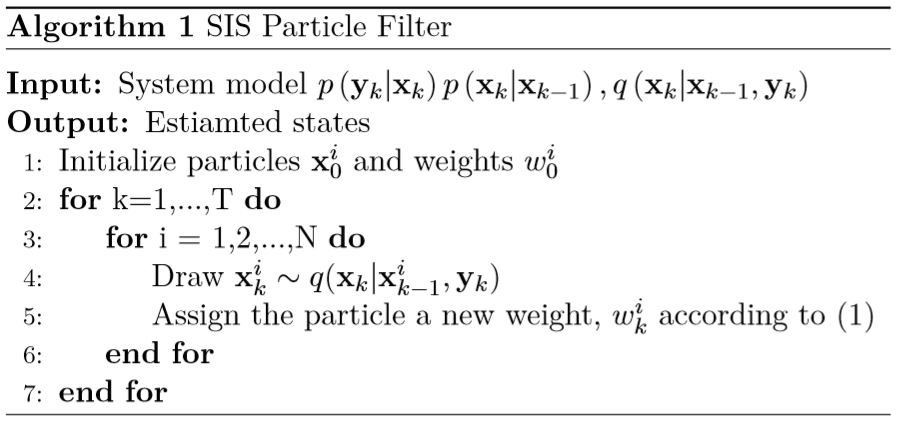总结
这篇文章主要是自己学习粒子滤波之后的一些理解，主要参考了文章 A Tutorial on Particle Filters for Online Nonlinear/Non-Gaussian Bayesian Tracking，但是这篇文章中是从 general 的情况开始讲，然后举了一些常用的特例，个人感觉不利于初学者理解，因此本文写作过程中的思路是从简单的特例开始再到更一般的情况。
最后一部分 [粒子滤波原理推导](## 粒子滤波原理推导) 其实写的并没有很清楚，主要是因为自己太懒，到最后懒得一个个手敲公式了，如果想更清楚地了解其中的细节，可以阅读上面那篇论文。
Reference
M. S. Arulampalam, S. Maskell, N. Gordon and T. Clapp, “A tutorial on particle filters for online nonlinear/non-Gaussian Bayesian tracking,” in IEEE Transactions on Signal Processing, vol. 50, no. 2, pp. 174-188, Feb. 2002.
展开全文• 分析了无线移动传感器网络中目标的跟踪原理，研究了基本粒子滤波算法的主要技术。对基本粒子滤波的重要性函数和重采样技术进行改进后，给出了一种提高基本粒子滤波算法跟踪精度的方法。通过仿真比较可以看出改进粒子...
• ## 粒子滤波算法

千次阅读 2019-05-16 18:14:03
粒子滤波(PF: Particle Filter)的思想基于蒙特卡洛方法(Monte Carlo methods)，它是利用粒子集来表示概率，可以用在任何形式的状态空间模型上。其核心思想是通过从后验概率中抽取的随机状态粒子来表达其分布，是一种...
粒子滤波(PF: Particle Filter)的思想基于蒙特卡洛方法(Monte Carlo methods)，它是利用粒子集来表示概率，可以用在任何形式的状态空间模型上。其核心思想是通过从后验概率中抽取的随机状态粒子来表达其分布，是一种顺序重要性采样法(Sequential Importance Sampling)。简单来说，粒子滤波法是指通过寻找一组在状态空间传播的随机样本对概率密度函数进行近似，以样本均值代替积分运算，从而获得状态最小方差分布的过程。这里的样本即指粒子,当样本数量N→∝时可以逼近任何形式的概率密度分布。
一、步骤
t = 0时，粒子初始化。随机生成粒子集并设置权值。  t = 1, 2, ...， 重复以下步骤： a. 预测。根据系统的预测过程预测各个粒子的状态。 b. 更新。根据观测值更新粒子权值。 c. 重采样。复制一部分权值高的粒子，同时去掉一部分权值低的粒子。 d. 输出：状态估计。使用粒子和权值估计当前的状态。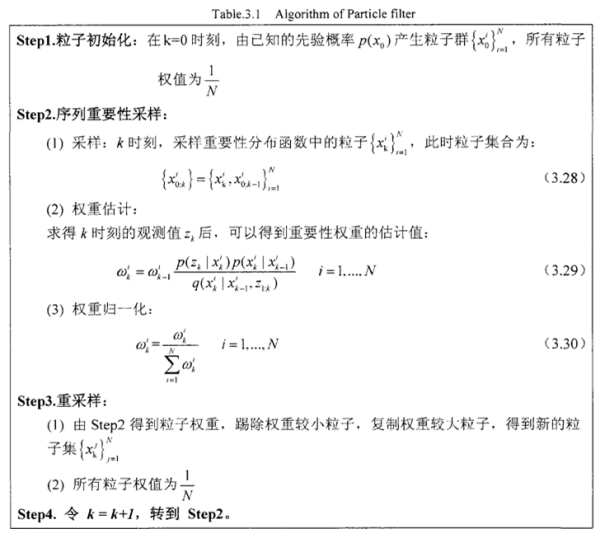二、概念
1、蒙特卡洛采样：根据待估计的量X的概率分布（后验概率）进行采样。
2、蒙特卡洛方法：在采集了N个样本之后，如果要求X的均值，那么可以直接对采集的N个样本 求平均计算出的值来代替样本的均值，当N足够大的时候，平均值的与均值的近似度越高，误差越小。
3、重要性采样：根据一个已知的分布去采样，就有期望为：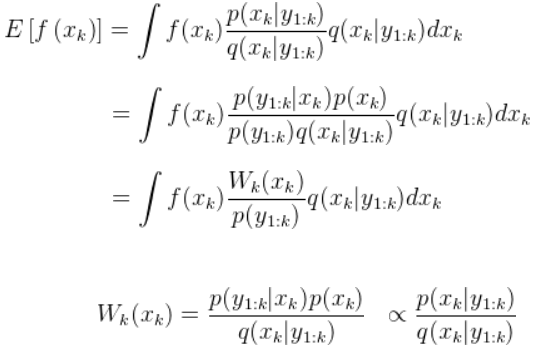重要的是引入了权重的概念，上面Wk(Xk)就是采样点的权重，引入权重之后，根据蒙特卡洛方法求均值就变为如下：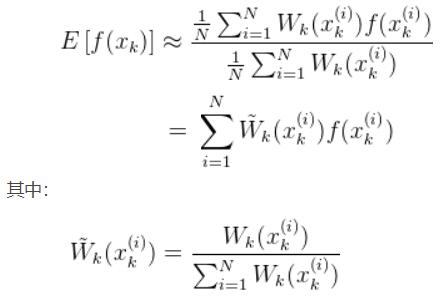所以引入重要性采样之后，权重经过了归一化处理（权重的和为1），根据权重最终求出了该随机量X的均值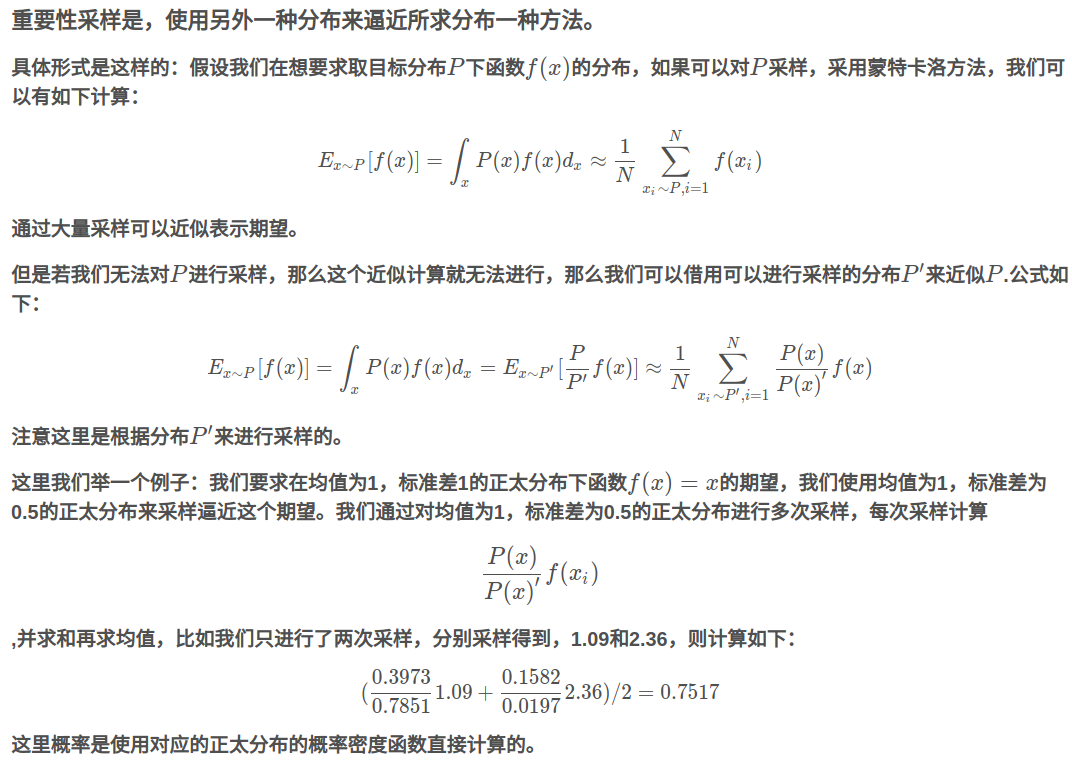展开全文状态估计 蒙特卡洛 重要性采样
• 粒子滤波算法对数学，尤其是概率论与数理统计知识要求很高。学好粒子滤波必须对其底层推导有深刻认识，所以需要有教科书式的指导，而论文综述往往太过笼统，无法将实际和理论数学联系起来，这本书很好。
• 一直都觉得粒子滤波是个挺牛的东西，每次试图看文献都被复杂的...从代码入手，一下子就明白了粒子滤波原理。根据维基百科上对粒子滤波的介绍(http://en.wikipedia.org/wiki/Particle_filter)，粒子滤波其实有很多...
• 实现了粒子滤波过程，包括状态预测，量测更新，粒子权重的计算，重采样，Roughening(粗糙处理），后验均值和方差。
• 粒子滤波算法中,重采样的引入有效地改善粒子退化现象,但同时也导致了粒子多样性减弱问题的产 生.本文给出了一种基于进化采样的改进粒子滤波算法.该算法在重采样过程后,首先根据马尔可夫链蒙特卡罗(Markov-Chain-...
• 1. 写在前面最近在看视频跟踪方面的一些硕博士毕业论文，几乎看到的每一篇都会涉及到粒子滤波算法，所以这段时间花了很多时间在看相关的内容。浏览了大量的博客和文章，跟着不停推导公式，感觉还是无法完全掌握，...
• 粒子滤波粒子滤波粒子滤波粒子滤波粒子滤波算算
• 在前一个博客中已经对机器视觉及粒子滤波算法的相关原理进行了介绍，在基于410c平台实现粒子滤波算法的前期，我们在上位机上应用QT和Opencv完成了粒子滤波算法的实现和测试。qt linux opencv
• 目标跟踪应用非常广泛，而是粒子滤波算法是众多跟踪算法中还算可以的一种，粒子滤波是一种序列蒙特卡罗滤波方法，其实质是利用一系列随机抽取的样本（即粒子）来替代状态的后验概率分布。在此不打算介绍和推理繁杂的...目标跟踪 运动跟踪 颜色直方图
• 粒子滤波是一种基于蒙特卡罗和递推贝叶斯估计...在本文中，介绍了粒子滤波基本原理，通过改进权重计算、重采样算法，计算速度得到提高。这种改进的算法在DSP系统中进行目标跟踪仿真，证明其具有速度快、精度高的特点。
• 转自：http://blog.csdn.net/sinat_31135199/article/details/55262359 ... 一直都觉得粒子滤波是个挺牛的东西，每次试图看文献都被复杂的数学符号搞得看不下去。一个偶然的机会发现了Rob Hess(ht
• tf为时间长度，k可以理解为时间轴上的k时刻；...生成100个粒子并根据预测和观测值差值计算各个粒子的权重；重采样对低权重进行剔除，同时保留高权重，防止退化的办法。经本人修改验证，可以下载研究。...